Next: 6. Negative Bias Temperature Up: 5. Dielectric Degradation and Previous: 5.2 Dielectric Wearout and

Subsections

# 5.3 Quantum Mechanical Tunneling

Figure 5.4: Energy band diagrams showing tunneling mechanisms in an MOS structure. (a) At direct tunneling conditions the electron tunnels through the whole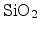energy barrier. (b) At Fowler-Nordheim conditions the electron tunnels through a part of the barrier to the conduction band of the insulator. From there it can flow to the anode.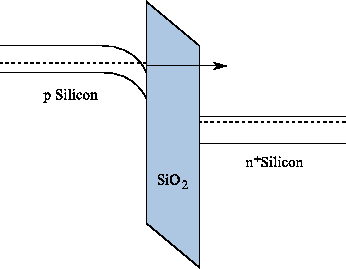Direct tunnelingFowler-Nordheim tunneling
Due to constant downscaling of gate-dielectric thicknesses in modern MOS devices the effect of tunneling has drastically gained relevance. Quantum mechanical tunneling describes the transition of carriers through a classically forbidden energy state. This can be an electron tunneling from the semiconductor through a dielectric, which represents an energy barrier, to the gate contact of an MOS structure. Even if the energy barrier is higher than the electron energy, there is quantum mechanically a finite probability of this transition. The reason lies in the wavelike behavior of particles on the quantum scale where the wave function describes the probability of finding an electron at a certain position in space. As the wave function penetrates the barrier and can even extend to the other side, quantum mechanics predict a non-zero probability for an electron to be on the other side.

Figure 5.4 depicts the energy band diagrams for two tunneling mechanisms in an MOS structure consisting of a p-type bulk silicon,dielectric, and a npolycrystalline silicon gate.

## 5.3.1 Direct Tunneling

In Figure 5.4(a) the energy band conditions for the direct tunneling regime are shown. Here, the electrons from the inverted silicon surface can tunnel directly through the forbidden energy barrier formed by the dielectric layer to the poly-gate. Direct tunneling is strongly gaining significance when the dielectric layer gets thinner.

A common approach to model the tunneling current is the Tsu-Esaki formula(5.1)

where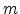is the electron mass in silicon and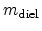the electron mass in the dielectric .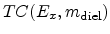is the transmission coefficient and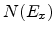the supply function which is given as(5.2)

Here,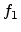anddenote the energy distribution functions near the interfaces .

### 5.3.1.1 Fowler-Nordheim Tunneling

The energy band conditions for Fowler-Nordheim tunneling, which is a special case of direct tunneling, are depicted in Figure 5.4(b). The electrons do not tunnel directly to the other side of the barrier. Instead they tunnel from the silicon inversion layer to the conduction band of thelayer from where they are transported to the gate contact. The Fowler-Nordheim regime is significant for thicker dielectrics and sufficiently high electric fields.

A thorough investigation of modeling and simulation of tunneling mechanisms can be found in the thesis of Gehring .

## 5.3.2 Trap-Assisted Tunneling

As the reduction of the applied voltages does not keep up with the miniaturization of actual devices the electric fields across dielectric layers are constantly increasing. Especially for non-volatile memory cells high electric fields are necessary in order to achieve quick write and erase cycles. Due to the repeated high-field stress, defects can arise in the dielectric leading to tunneling currents, even at low fields. This stress-induced leakage current (SILC) [70,71] plays a major role in the determination of the retention times of non-volatile memory cells.

There are many approaches to model trap-assisted tunneling (TAT). One is to model the defect assisted tunneling process including a single trap. For each trap position tunneling from the cathode to the trap and further to the anode is considered. From this the trap occupancy function can be calculated which is used to compute the tunneling current . This single-TAT approach works very well for slightly degraded devices or devices with thin gate dielectrics. For thicker dielectrics with a high defect density it is reasonable to assume that also the interaction of two or more traps in the tunneling process takes place .

For the modeling of multi-trap assisted tunneling (multi-TAT) approaches like a two-trap process [74,75] or a multi-trap process considering hopping of carriers between distinct defects  have been presented. Recently, anomalous charge loss in floating-gate memory cells has been reported , where a two-trap model was used to reproduce the measured data.

For correct modeling of such highly degraded devices a new approach is proposed as part of this work. It rigorously computes TAT current assisted by multiple traps [77,78]. In this model hopping processes between all oxide defects are taken into account. In addition the filling of oxide traps with carriers, leading to space charge in the oxide and therefore to a shift of the threshold voltage, is accounted for. This shift can lead to circuit failure as the timing parameters of the device are degraded.

The model for the simulation of SILC in highly degraded devices is based on inelastic, phonon-assisted tunneling  with the tunneling-rate proposed by Herrmann and Schenk . These approaches are extended for modeling the interaction of multiple traps in the tunneling process.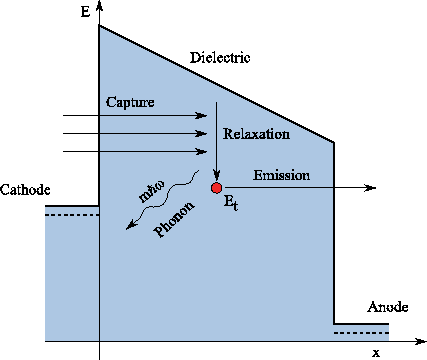### 5.3.2.1 Inelastic Phonon-Assisted Tunneling

The defect-assisted tunneling process of an electron from the cathode to the anode via a trap is considered as a two-step process. Electrons are captured from the cathode, relax to the energy level of the trap by emitting one or more phonons with the energy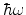, and are then emitted to the anode. This process is inelastic as the electron energy is not conserved during the tunneling process. Figure 5.5 depicts this process including the phonon emission.

### 5.3.2.2 Single-Trap Assisted Tunneling

In the single-trap assisted tunneling approach the tunneling current for each trap is calculated separately. The total tunneling current is afterwards superimposed from the individual contributions. Although the interaction between neighboring traps is of importance in highly degraded dielectrics (Section 5.3.2.3), for less degraded devices this approach can be used [72,80].

The tunneling current density is modeled as the sum of capture or emission rates of each trap,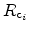or, which are equal in the stationary case,, multiplied by the trap cross section,(5.3)

The energetic position of the trap,, with respect to the conduction band edge determines the trap cross section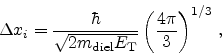(5.4)

wheredenotes the electron mass in the dielectric, which is used as a model calibration parameter.

The single-TAT and the multi-TAT models differ in the way the capture and emission rates are calculated. When only single-trap processes are considered (see Figure 5.5) the rates are determined by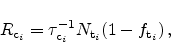(5.5)(5.6)

Here,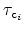and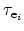are the capture and emission times and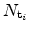is the trap concentration. As the capture and emission rates are equal in the stationary case, the trap occupancycan be directly calculated as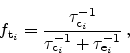(5.7)

where the inverse capture and emission times are defined as as [72,82](5.8)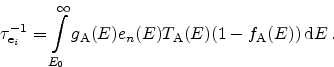(5.9)

In these expressions,and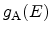denote the density of states in the cathode and anode, respectively,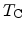and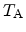the transmission coefficients from the cathode and the anode, and the symbolsand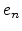are computed as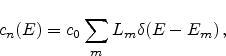(5.10)(5.11)

with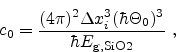(5.12)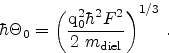(5.13)

The summation indexgives the number of discrete phonon emissions,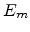is the phonon energy, andis the multiphonon transition probability . The symbols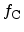andrepresent the Fermi-distributions,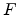the electric field in the dielectric, and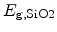the band gap of. The transmission coefficients were evaluated by a numerical WKB method which yields reasonable accuracy for single-layer dielectrics. This model has been used in a more or less similar form by various authors [80,79,82].### 5.3.2.3 Multi-Trap Assisted Tunneling

For highly degraded devices the isolated calculation for each trap is not sufficient anymore . Anomalous charge loss in memory cells has been observed and was explained by conduction through a second trap . The single-trap model can be extended for this case, and the rate equations become (see Figure 5.6)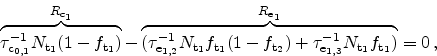(5.14)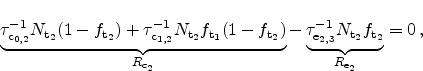(5.15)

where instantaneous transitions between occupied and free traps are assumed.

For thicker dielectrics it is quite reasonable to assume that an arbitrary number of traps assist in the conduction process. We therefore extend the model to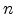traps where the capture and emission rates are evaluated as(5.16)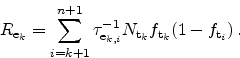(5.17)

The values for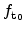and, which are the trap occupation probabilities at the cathode and the anode, are set to 1 and 0, respectively. This way the cathode acts as a perfect electron source and the anode as an electron sink.

From the capture and emission rates the following equation system can be set up(5.18)

from which the values of all trap occupation probabilities have to be calculated. This is performed using a Newton method with the cost functionfor a trap at position(5.19)

and the values of the derivatives in the Jacobian matrix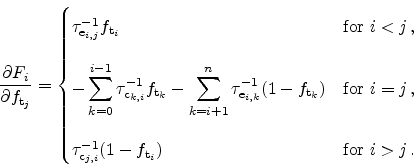(5.20)

A typical number of unknowns of the equation system is 15. Depending on the dielectric thickness and trap energy the result does not improve when further increasing this number. The computational effort remains negligible compared to the total device simulation time. The multi-trap assisted tunneling current density can then be obtained from the capture or emission rates(5.21)

### 5.3.2.4 Influence on the Threshold Voltage

By coupling this model to the semiconductor device equations (Section 2.1) the simulation of the effect of charged defects on the threshold voltage of memory devices is possible. The space charge density in the dielectric is calculated from the trap occupation function as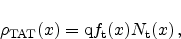(5.22)

and added to Poisson's equation as described in Section 2.1.2 with(5.23)

The trapped electrons shift the threshold voltage to more positive voltages and can lead to circuit failure.

## 5.3.3 Evaluation of the New Model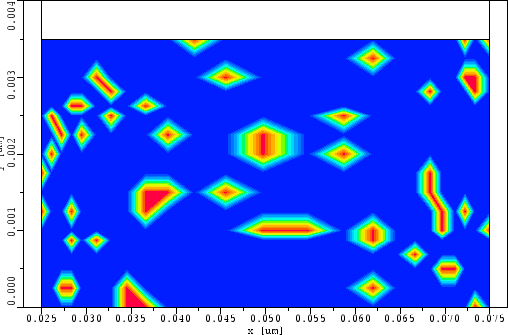The implementation of these models into the device- and circuit-simulator Minimos-NT  allows the two- and three-dimensional study of single- and multi-trap assisted tunneling as well as direct tunneling mechanisms. Figure 5.7 gives an example of a highly degraded dielectric with traps randomly distributed across the oxide. The dielectric can now be used for the simulation of multi-trap assisted tunneling.

Figure 5.8: (a) SILC simulations for a set of MOS devices at 1 V gate bias. The oxide was assumed to have a constant trap concentration. The multi-TAT current is always larger than the single-TAT current. (b) The trap occupancy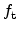in the oxide of a 1.5 nm MOS transistor at 1 V gate bias.Oxide thickness variation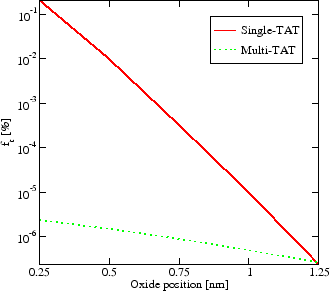Trap occupancy along the channel

Figure 5.8(a) shows a comparison of SILC simulation results assuming three different tunneling mechanisms, namely direct tunneling, single-TAT, and multi-TAT. The models have been applied to a set of MOS transistors with gate dielectric thicknesses ranging from 1.5nm up to 9nm. The gate is biased at 1V, source and drain are kept at 0V. For both, the single-TAT and the multi-TAT simulations, the trap energy is set to 2.8eV below the dielectric conduction band with a constant trap density of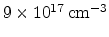across the oxide.

In the multi-trap simulation the tunneling current is several orders of magnitude higher than in the single-trap simulation. This is due to the fact that the multi-TAT current includes the single-TAT component as a limiting case. The multi-TAT model considers the capture and emission processes from the cathode and to the anode, respectively, and also the capture and emission processes involving all other trap centers. This leads to the comparably high multi-TAT component in devices with thicker oxides. It has to be considered, though, that this high current is mainly due to the assumption of uniformly distributed trap concentrations across the oxide, which implies that the total amount of traps is much higher in thick dielectrics. The direct tunneling component loses importance for thicker dielectrics but dominates for thin dielectrics as found in logic CMOS devices. For devices with thicker oxides and higher trap densities multi-TAT processes become increasingly important.

Figure 5.8(b) depicts the resulting trap occupancy within the oxide. An MOS transistor with 1V gate bias was simulated. It can be seen that the trap occupancyis remarkably lower in the multi-TAT case. The reason is the higher probability for electrons to tunnel to one of the neighbor traps compared to tunneling to the anode as it is the only possibility in the single-TAT model.

Figure 5.9 outlines the threshold voltage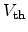for different oxide thicknesses. The direct tunneling model, applying the commonly used Tsu-Esaki approach, does not account for the filling of traps in the oxide. Therefore the threshold voltage is not shifted compared to the simulation without a tunneling model. The new multi-TAT model predicts an increase in. This higher threshold voltage is due to the filled and therefore negatively charged traps.

For thicker dielectrics with high defect density the inclusion of multiple traps is crucial for the simulation of both, quantum mechanical gate currents and the shift of threshold voltages. Thin dielectrics, on the other hand side, may be as well treated using the single-TAT model.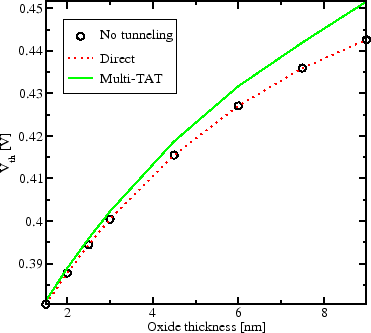Next: 6. Negative Bias Temperature Up: 5. Dielectric Degradation and Previous: 5.2 Dielectric Wearout and

R. Entner: Modeling and Simulation of Negative Bias Temperature Instability